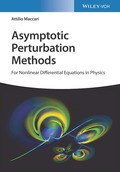Wiley-VCH, Berlin Asymptotic Perturbation MethodsThe book presents a unified view on nonlinear systems in physics by providing a common framework to .. Product #: 978-3-527-41421-5 Regular price: \$120.56 \$120.56 Auf Lager

# Asymptotic Perturbation Methods

## For Nonlinear Differential Equations in Physics1. Auflage Februar 2023
256 Seiten, Hardcover
83 Abbildungen
Monographie

ISBN: 978-3-527-41421-5
Wiley-VCH, Berlin

### Kurzbeschreibung

The book presents a unified view on nonlinear systems in physics by providing a common framework to obtain approximate solutions to the respective nonlinear partial differential equations based on the asymptotic perturbation method.

Jetzt kaufen

### Preis: 129,00 €

Preis inkl. MwSt, zzgl. Versand

1 THE ASYMPTOTIC PERTURBATION METHOD FOR NONLINEAR OSCILLATORS
1.1 Introduction
1.2 Nonlinear Dynamical Systems
1.3 The Approximate Solution
1.4 Comparison with the Results of the Numerical Integration
1.5 External Excitation in Resonance with the Oscillator
1.6 Conclusion
2 THE ASYMPTOTIC PERTURBATION METHOD FOR REMARKABLE NONLINEAR OSCILLATORS
2.1 Introduction
2.2 Periodic Solutions and their Stability
2.3 Global Analysis of the Model System
2.4 Infinite-Period Symmetric Homoclinic Bifurcation
2.5 A Few Considerations
2.6 A Peculiar Quasiperiodic Attractor
2.7 Building an Approximate Solution
2.8 Results from Numerical Simulation
2.9 Conclusion
3 THE ASYMPTOTIC PERTURBATION METHOD FOR VIBRATION CONTROL WITH TIME DELAY STATE FEEDBACK
3.1 Introduction
3.2 Time Delay State Feedback
3.3 The AP Method
3.4 Stability Analysis and Parametric Resonance Control
3.5 Suppression of the Two-Period Quasiperiodic Motion
3.6 Vibration Control for Other Nonlinear Systems
4 THE ASYMPTOTIC PERTURBATION METHOD FOR VIBRATION CONTROL WITH NONLOCAL DYNAMICS
4.1 Introduction
4.2 Vibration Control for the van der Pol Equation
4.3 Stability Analysis and Parametric Resonance Control
4.4 Suppression of the Two-Period Quasiperiodic Motion
4.5 Conclusion
5 THE ASYMPTOTIC PERTURBATION METHOD FOR NONLINEAR CONTINUOUS SYSTEMS
5.1 Introduction
5.2 The Approximate Solution for the Primary Resonance of the nth Mode
5.3 The Approximate Solution for the Subharmonic Resonance of Order One-Half of the nth Mode
5.4 Conclusion
6 THE ASYMPTOTIC PERTURBATION METHOD FOR DISPERSIVE NONLINEAR PARTIAL DIFFERENTIAL
EQUATIONS
6.1 Introduction
6.2 Model Nonlinear PDEs Obtained from the Kadomtsev-Petviashvili Equation
6.3 The Lax Pair for the Model Nonlinear PDE
6.4 A Few Considerations
6.5 A Generalized Hirota Equation in 2+1 dimensions
6.6 Model Nonlinear PDEs Obtained from the KP equation
6.7 The Lax Pair for the Hirota-Maccari Equation
6.8 Conclusion
7 THE ASYMPTOTIC PERTURBATION METHOD FOR PHYSICS PROBLEMS
7.1 Introduction
7.2 Derivation of the Model System
7.3 Integrability of the Model System of Equations
7.4 Exact Solutions for the C-Integrable Model Equation
7.5 Conclusion
8 THE ASYMPTOTIC PERTURBATION METHOD FOR ELEMENTARY PARTICLE PHYSICS
8.1 Introduction
8.2 Derivation of the Model System
8.3 Integrability of the Model System of Equations
8.4 Exact solutions for the C-Integrable Model Equation
8.5 A Few Considerations
8.6 Hidden Symmetry Models
8.7 Derivation of the Model System
8.8 Coherent Solutions
8.9 Chaotic and Fractal Solutions
8.10 Conclusion
9 THE ASYMPTOTIC PERTURBATION METHOD FOR ROGUE WAVES IN NONLINEAR SYSTEMS
9.1 Introduction
9.2 The Mathematical Framework
9.3 The Maccari System
9.4 Rogue Waves Physical Explanation according to Maccari System and Blowing Solutions
9.5 Conclusion
10 THE ASYMPTOTIC PERTURBATION METHOD FOR FRACTAL AND CHAOTIC SOLUTIONS IN NONLINEAR PARTIAL DIFFERENTIAL EQUATIONS
10.1 Introduction
10.2 A new Integrable System from the Dispersive Long Wave Equation
10.3 Nonlinear Coherent Solutions
10.4 Chaotic and Fractal Solutions
10.5 Conclusion
11 THE ASYMPTOTIC PERTURBATION METHOD FOR NONLINEAR QUANTUM MECHANICS
11.1 Introduction
11.2 The NLS equation for a1>0
11.3 The NLS equation for a1<0
11.4 A Possible Extension
11.5 The Nonrelativistic Case
11.6 The Relativistic Case
11.7 Conclusion
12 COSMOLOGY
12.1 Introduction
12.2 A New Field Equation
12.3 Exact solution in the Robertson-Walker Metrics
12.4 Entropy Production
12.5 Conclusion
13 CONFINEMENT AND ASYMPTOTIC FREEDOM IN A PURELY GEOMETRIC FRAMEWORK
13.1 Introduction
13.2 The Uncertainty Principle
13.3 Confinement and Asymptotic Freedom for the Strong Interaction
13.4 The Motion of a Light Ray into a Hadron
13.5 Conclusion
14 THE ASYMPTOTIC PERTURBATION METHOD FOR A REVERSE INFINITE-PERIOD BIFURCATION IN THE NONLINEAR SCHRÖDINGER EQUATION
14.1 Introduction
14.2 Building an Approximate Solution
14.3 A Reverse Infinite-Period Bifurcation
14.4 Conclusion
Conclusion
Bibliography
Attilio Maccari is Associate Professor of Physics at the Technical Institute Cardano in Rome, Italy. Ever since his PhD his research is focused on nonlinear partial differential equations in physics and their solutions using asymptotic perturbation methods.